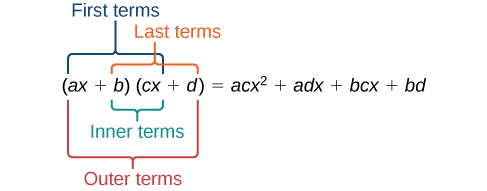## Sums and Products of Polynomials

### Learning Outcomes

• Multiply and divide polynomials

We can add and subtract polynomials by combining like terms which are terms that contain the same variables raised to the same exponents. For example, $5{x}^{2}$ and $-2{x}^{2}$ are like terms and can be added to get $3{x}^{2}$, but $3x$ and $3{x}^{2}$ are not like terms; therefore, they cannot be added.

### Example

Find the sum.

$\left(12{x}^{2}+9x - 21\right)+\left(4{x}^{3}+8{x}^{2}-5x+20\right)$

Here is a summary of some helpful steps for adding and subtracting polynomials.

### How To: Given multiple polynomials, add or subtract them to simplify the expressions

1. Combine like terms.
2. Simplify and write in standard form.

When you subtract polynomials, you will still be looking for like terms to combine, but you will need to pay attention to the sign of the terms you are combining. In the following example we will show how to distribute the negative sign to each term of a polynomial that is being subtracted from another.

### Example

Find the difference.

$\left(7{x}^{4}-{x}^{2}+6x+1\right)-\left(5{x}^{3}-2{x}^{2}+3x+2\right)$

## Multiplying Polynomials

Multiplying polynomials is a bit more challenging than adding and subtracting polynomials. We must use the distributive property to multiply each term in the first polynomial by each term in the second polynomial. We then combine like terms.

You may have used the distributive property to help you solve linear equations such as $2\left(x+7\right)=21$. We can distribute the $2$ in $2\left(x+7\right)$ to obtain the equivalent expression $2x+14$. When multiplying polynomials, the distributive property allows us to multiply each term of the first polynomial by each term of the second. We then add the products together and combine like terms to simplify.

The following video will provide you with examples of using the distributive property to find the product of monomials and polynomials.

Below is a summary of the steps we used to find the product of two polynomials using the distributive property.

### How To: Given the multiplication of two polynomials, use the distributive property to simplify the expression

1. Multiply each term of the first polynomial by each term of the second.
2. Combine like terms.
3. Simplify.

## Using FOIL to Multiply Binomials

We can also use a shortcut called the FOIL method when multiplying two binomials. It is called FOIL because we multiply the first terms, the outer terms, the inner terms, and then the last terms of each binomial.The FOIL method arises out of the distributive property. We are simply multiplying each term of the first binomial by each term of the second binomial and then combining like terms.

### Example

Use FOIL to find the product. $(2x-18)(3x+3)$

In the following video, we show an example of how to use the FOIL method to multiply two binomials.

The following steps summarize the process for using FOIL to multiply two binomials. It is very important to note that this process only works for the product of two binomials. If you are multiplying a binomial and a trinomial, it is better to use a table to keep track of your terms.

### How To: Given two binomials, use FOIL to simplify the expression

1. Multiply the first terms of each binomial.
2. Multiply the outer terms of the binomials.
3. Multiply the inner terms of the binomials.
4. Multiply the last terms of each binomial.